# height2range

Convert target height to propagated range

## Syntax

r = height2range(tgtht,anht,el)
r = height2range(tgtht,anht,el,Name=Value)
[r,trueSR,trueEL] = height2range(___,Method="CRPL")

## Description

example

r = height2range(tgtht,anht,el) returns the propagated range to the target, r, as a function of the target height tgtht, the sensor height anht, and the local elevation angle el assuming a Curved Earth Model with a 4/3 effective Earth radius.

example

r = height2range(tgtht,anht,el,Name=Value) specifies additional inputs using name-value arguments. For example, you can specify a flat Earth model, a curved Earth model with a given radius, or a CRPL Exponential Reference Atmosphere Model with custom values.
[r,trueSR,trueEL] = height2range(___,Method="CRPL") also returns the true slant range and the true elevation angle when you specify the Earth model as "CRPL".

## Examples

collapse all

Compute the range along the propagated path for a target height of 1 km, an antenna height of 10 meters, and an elevation angle of 2 degrees at the radar. Assume a curved Earth model with a 4/3 effective Earth radius.

r = height2range(1e3,10,2)
r = 2.7125e+04 

Compute the range along the propagated path using the CRPL exponential reference atmosphere. Assume a target height of 1 km, an antenna height of 10 meters, and an elevation angle of 2 degrees at the radar. Additionally, compute the true slant range and the true elevation angle to the target.

[R,SRtrue,elTrue] = height2range(1e3,10,2,Method="CRPL")
R = 2.7171e+04 
SRtrue = 2.7163e+04 
elTrue = 1.9666 

## Input Arguments

collapse all

Target height in meters, specified as a nonnegative real-valued scalar or vector. If tgtht is a vector, it must have the same size as the other vector input arguments of height2range. Heights are referenced to the ground.

Data Types: double

Sensor height in meters, specified as a nonnegative real-valued scalar or vector. If anht is a vector, it must have the same size as the other vector input arguments of height2range. Heights are referenced to the ground.

Data Types: double

Local elevation angle in degrees, specified as a real-valued scalar or vector. The local elevation angle is the initial elevation angle of the ray leaving the sensor. If el is a vector, it must have the same size as the other vector input arguments of height2range.

Data Types: double

### Name-Value Arguments

Specify optional pairs of arguments as Name1=Value1,...,NameN=ValueN, where Name is the argument name and Value is the corresponding value. Name-value arguments must appear after other arguments, but the order of the pairs does not matter.

Example: Method="CRPL",SurfaceRefractivity=300,RefractionExponent=0.15

Earth model used for the computation, specified as "Curved", "Flat", or "CPRL".

Data Types: char | string

Effective Earth radius in meters, specified as a positive scalar. If this argument is not specified, height2range calculates the effective Earth radius using a refractivity gradient of –39 × 10–9 N-units/meter, which results in approximately 4/3 of the real Earth radius. This argument applies only if Method is specified as "Curved".

Data Types: double

Surface refractivity in N-units, specified as a nonnegative real-valued scalar. The surface refractivity is a parameter of the CRPL Exponential Reference Atmosphere Model used by height2range. This argument applies only if Method is specified as "CRPL".

Data Types: double

Refraction exponent, specified as a nonnegative real-valued scalar. The refraction exponent is a parameter of the CRPL Exponential Reference Atmosphere Model used by height2range. This argument applies only if Method is specified as "CRPL".

Data Types: double

## Output Arguments

collapse all

Propagated range between the target and the sensor in meters, returned as a real-valued scalar or row vector. If r is a vector, it has the same size as the vector input arguments of height2range.

True slant range in meters, returned as a real-valued scalar or row vector. If trueSR is a vector, it has the same size as the vector input arguments of height2range. This argument is available only if Method is specified as "CRPL".

True elevation angle in degrees, returned as a real-valued scalar or row vector. If trueEL is a vector, it has the same size as the vector input arguments of height2range. This argument is available only if Method is specified as "CRPL".

collapse all

### Flat Earth Model

The flat Earth model assumes that the Earth has infinite radius and that the index of refraction of air is uniform throughout the atmosphere. The flat Earth model is applicable over short distances and is used in applications like communications, automotive radar, and synthetic aperture radar (SAR).

Given the antenna height ha and the initial elevation angle θ0, the model relates the target height hT and the slant range RT by

${h}_{T}={h}_{a}+{R}_{T}\mathrm{sin}{\theta }_{0}\text{ }⇔\text{ }{R}_{T}=\left({h}_{T}-{h}_{a}\right)\mathrm{csc}{\theta }_{0},$

so knowing one of those magnitudes enables you to compute the other. The actual range R is equal to the slant range. The true elevation angle θT is equal to the initial elevation angle.

To compute the ground range G, use

$G=\left({h}_{T}-{h}_{a}\right)\mathrm{cot}{\theta }_{0}.$### Curved Earth Model

The fact that the index of refraction of air depends on height can be treated approximately by using an effective Earth's radius larger than the actual value.

Given the effective Earth's radius R0, the antenna height ha, and the initial elevation angle θ0, the model relates the target height hT and the slant range RT by

${\left({R}_{0}+{h}_{T}\right)}^{2}={\left({R}_{0}+{h}_{a}\right)}^{2}+{R}_{T}^{2}+2{R}_{T}\left({R}_{0}+{h}_{a}\right)\mathrm{sin}{\theta }_{0},$

so knowing one of those magnitudes enables you to compute the other. In particular,

${h}_{T}=\sqrt{{\left({R}_{0}+{h}_{a}\right)}^{2}+{R}_{T}^{2}+2{R}_{T}\left({R}_{0}+{h}_{a}\right)\mathrm{sin}{\theta }_{0}}-{R}_{0}.$

The actual range R is equal to the slant range. The true elevation angle θT is equal to the initial elevation angle.

To compute the ground range G, use

$G={R}_{0}\varphi ={R}_{0}\mathrm{arcsin}\frac{{R}_{T}\mathrm{cos}{\theta }_{0}}{{R}_{0}+{h}_{T}}.$A standard propagation model uses an effective Earth's radius that is 4/3 times the actual value. This model has two major limitations:

1. The model implies a value for the index of refraction near the Earth's surface that is valid only for certain areas and at certain times of the year. To mitigate this limitation, use an effective Earth's radius based on the near-surface refractivity value.

2. The model implies a value for the gradient of the index of refraction that is unrealistically low at heights of around 8 km. To partially mitigate this limitation, use an effective Earth's radius based on the platform altitudes.

For more information, see effearthradius.

### CRPL Exponential Reference Atmosphere Model

Atmospheric refraction evidences itself as a deviation in an electromagnetic ray from a straight line due to variation in air density as a function of height. The Central Radio Propagation Laboratory (CRPL) exponential reference atmosphere model treats refraction effects by assuming that the index of refraction n(h) and the refractivity N decay exponentially with height. The model defines

$N=\left(n\left(h\right)-1\right)×{10}^{6}={N}_{\text{s}}{e}^{-{R}_{\text{exp}}h},$

where Ns is the atmospheric refractivity value (in units of 10–6) at the surface of the earth, Rexp is the decay constant, and h is the height above the surface in kilometers. Thus

$n\left(h\right)=1+\left({N}_{\text{s}}\text{\hspace{0.17em}}×{10}^{-6}\right){e}^{-{R}_{\text{exp}}h}.$

The default value of Ns is 313 N-units and can be modified using the SurfaceRefractivity name-value argument in functions that accept it. The default value of Rexp is 0.143859 km–1 and can be modified using the RefractionExponent name-value argument in functions that accept it.

### CRPL Model Geometry

When the refractivity of air is incorporated into the curved Earth model, the ray paths do not follow a straight line but curve downward. (This statement assumes standard atmospheric propagation and nonnegative elevation angles.) The true elevation angle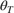is different from the initial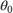. The actual range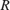, which is the distance along the curved path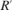, is different from the slant range.

Given the Earth's radius, the antenna height, the initial elevation angle, and the height-dependent index of refractionwith value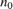at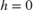, the modified model relates the target heightand the actual rangebyWhen Method is specified as "CRPL", the integral is solved usingfrom CRPL Exponential Reference Atmosphere Model.

To compute the ground range, use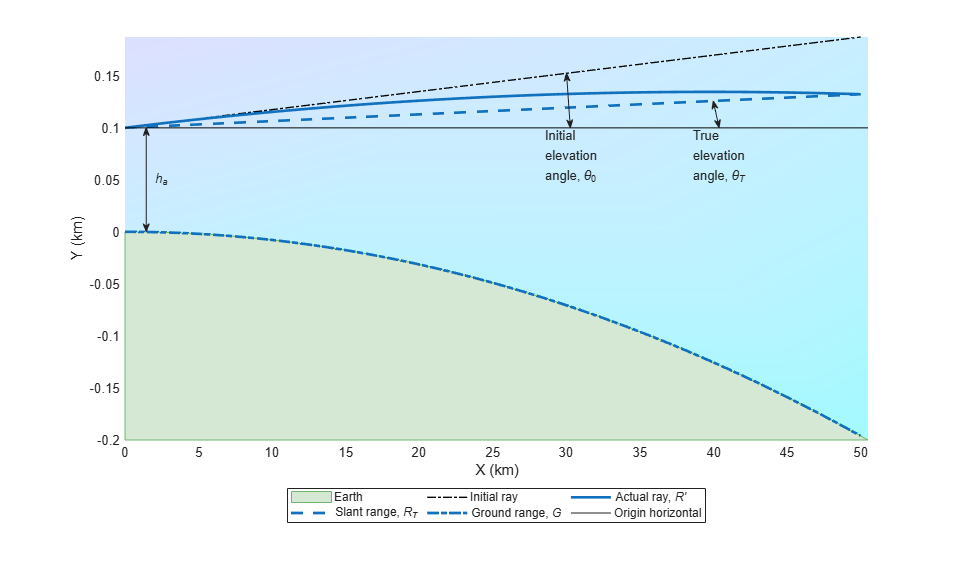## Version History

Introduced in R2021b